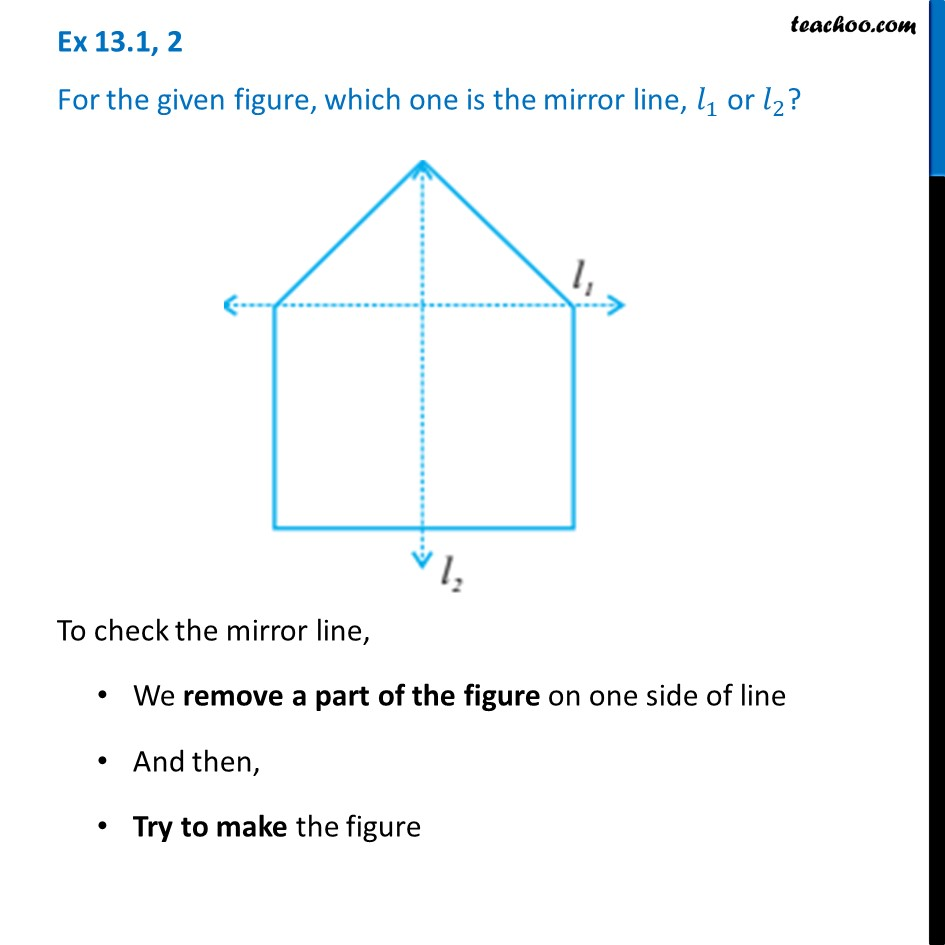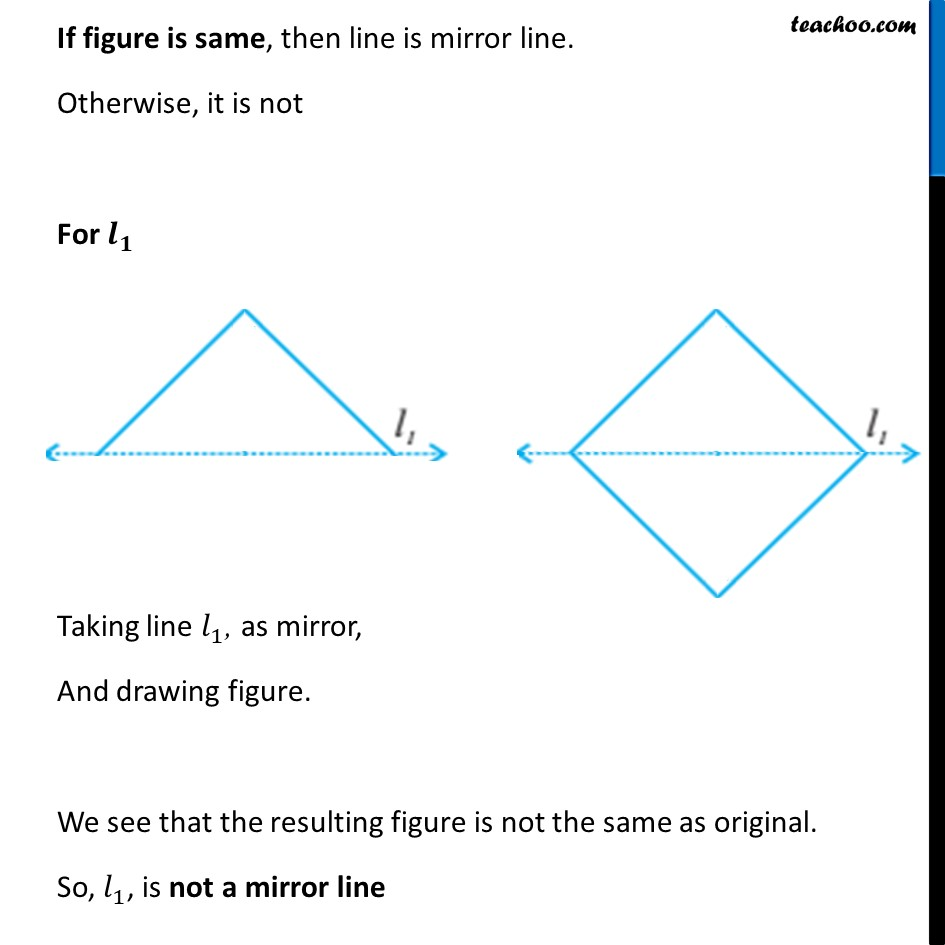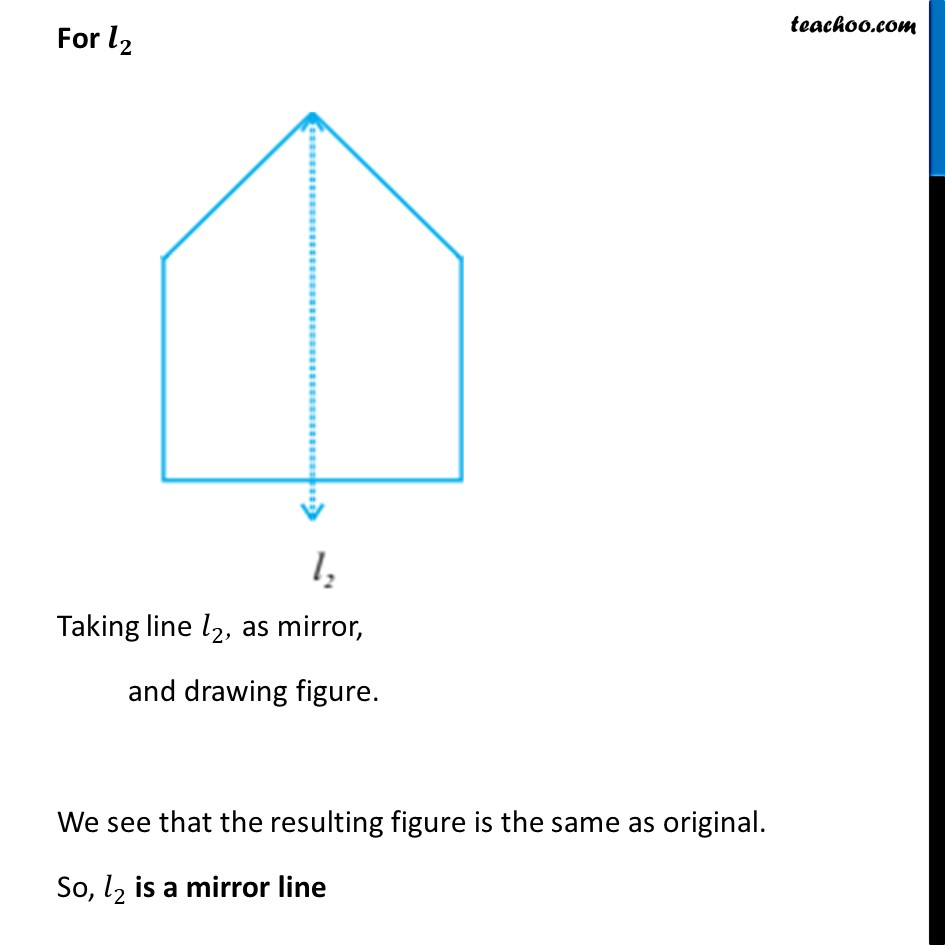Symmetry Definition

Symmetry
Serial order wiseLearn in your speed, with individual attention - Teachoo Maths 1-on-1 Class

### Transcript

Question 2 For the given figure, which one is the mirror line, 𝑙_1 or 𝑙_2?To check the mirror line, We remove a part of the figure on one side of line And then, Try to make the figure If figure is same, then line is mirror line. Otherwise, it is not For 𝒍_𝟏 Taking line 𝑙_1, as mirror, And drawing figure. We see that the resulting figure is not the same as original. So, 𝑙_1, is not a mirror line For 𝒍_𝟐 Taking line 𝑙_2, as mirror, and drawing figure. We see that the resulting figure is the same as original. So, 𝑙_2 is a mirror line